NetworkX

#### Previous topic

closeness_centrality

#### Next topic

edge_betweenness_centrality

# betweenness_centrality¶

betweenness_centrality(G, k=None, normalized=True, weight=None, endpoints=False, seed=None)

Compute the shortest-path betweenness centrality for nodes.

Betweenness centrality of a node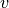is the sum of the fraction of all-pairs shortest paths that pass through: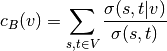where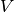is the set of nodes,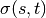is the number of shortest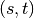-paths, and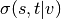is the number of those paths passing through some nodeother than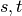. If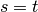,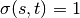, and if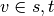,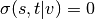[R155].

Parameters : G : graph A NetworkX graph k : int, optional (default=None) If k is not None use k node samples to estimate betweenness. The value of k <= n where n is the number of nodes in the graph. Higher values give better approximation. normalized : bool, optional If True the betweenness values are normalized by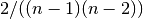for graphs, and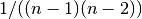for directed graphs where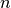is the number of nodes in G. weight : None or string, optional If None, all edge weights are considered equal. Otherwise holds the name of the edge attribute used as weight. endpoints : bool, optional If True include the endpoints in the shortest path counts. nodes : dictionary Dictionary of nodes with betweenness centrality as the value.

Notes

The algorithm is from Ulrik Brandes [R154]. See [R155] for details on algorithms for variations and related metrics.

For approximate betweenness calculations set k=#samples to use k nodes (“pivots”) to estimate the betweenness values. For an estimate of the number of pivots needed see [R156].

For weighted graphs the edge weights must be greater than zero. Zero edge weights can produce an infinite number of equal length paths between pairs of nodes.

References

 [R154] (1, 2) A Faster Algorithm for Betweenness Centrality. Ulrik Brandes, Journal of Mathematical Sociology 25(2):163-177, 2001. http://www.inf.uni-konstanz.de/algo/publications/b-fabc-01.pdf
 [R155] (1, 2, 3) Ulrik Brandes: On Variants of Shortest-Path Betweenness Centrality and their Generic Computation. Social Networks 30(2):136-145, 2008. http://www.inf.uni-konstanz.de/algo/publications/b-vspbc-08.pdf
 [R156] (1, 2) Ulrik Brandes and Christian Pich: Centrality Estimation in Large Networks. International Journal of Bifurcation and Chaos 17(7):2303-2318, 2007. http://www.inf.uni-konstanz.de/algo/publications/bp-celn-06.pdf